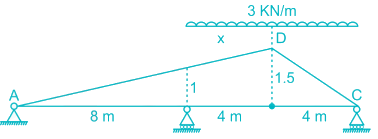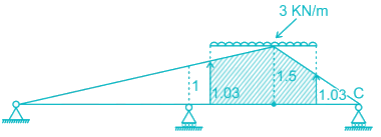Courses

# Structural Analysis - 2

## 10 Questions MCQ Test RRB JE for Civil Engineering | Structural Analysis - 2

Description
This mock test of Structural Analysis - 2 for Civil Engineering (CE) helps you for every Civil Engineering (CE) entrance exam. This contains 10 Multiple Choice Questions for Civil Engineering (CE) Structural Analysis - 2 (mcq) to study with solutions a complete question bank. The solved questions answers in this Structural Analysis - 2 quiz give you a good mix of easy questions and tough questions. Civil Engineering (CE) students definitely take this Structural Analysis - 2 exercise for a better result in the exam. You can find other Structural Analysis - 2 extra questions, long questions & short questions for Civil Engineering (CE) on EduRev as well by searching above.
QUESTION: 1

### Which one of the following structures is statically determinate and stable?

Solution:

A structure will be statically determinate if the external reactions can be determined from force-equilibrium equations. A structure is stable when the whole or part of the structure is prevented from large displacements on account of loading.

In option '1', the structure is stable and there are three reaction components which can be determined by two force equilibrium conditions and one-moment equilibrium condition.

The structure in option '2' is stable but statically indeterminate to the second degree.

The structure is shown in option '3'  has both reaction components coinciding with each other, so the moment equilibrium condition will never be satisfied and the structure will not be under equilibrium.

In option '4' the structure is not stable as a little movement in the horizontal direction leads to the large displacement and there is no resistance offered by the structure in the horizontal direction.

QUESTION: 2

### Determine the kinematic indeterminacy of the beam shown below: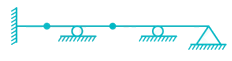Solution: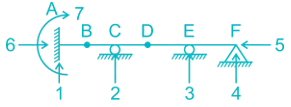Dk = 3J – re + rr

J = No. of joints

re = External support Reactions

rr = Released Reactions

re = 7

J = 6

rr = m – 1

m = No. of members meeting at internal hinge

At B:

rr = 2 – 1 = 1

At D:

rr = 2 – 1 = 1

Therefore,

Dk = 3 × 6 – 7 + 2

Dk = 13

QUESTION: 3

### The total degree of indeterminacy (external + internal) for the bridge truss is :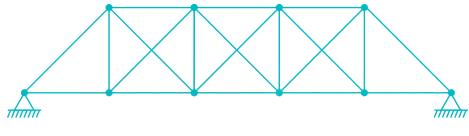Solution:

Dsi = m – (2j – 3)

m = 20

j = 10

Dsi = 20 – (2 × 10 – 3) = 3

Dse = re – 3 = 4 – 3 = 1

Total indeterminacy = 3 + 1 = 4

QUESTION: 4

A two hinged semi-circular arch of radius R carries a concentrated load W at the crown. The horizontal thrust is

Solution: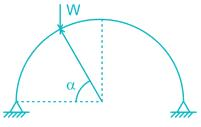H = W/π sin2α

If α = 90, H = W/π

QUESTION: 5

A three – hinged parabolic arch rid of span L and crown rise ‘h’ carries a uniformly distributed superimposed load of intensity ‘w’ per unit length. The hinges are located on two abutments at the same level and the third hinge at a quarter span location from the left-hand abutment. The horizontal trust on the abutment is:

Solution:

VA = VB = wL/2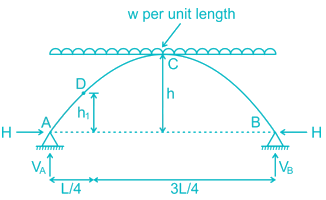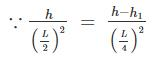⇒ 4 (h – h1) = h

3h = 4h1
aking moment about hinge at D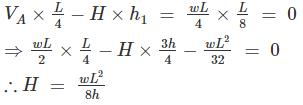QUESTION: 6

Static indeterminacy of the frame shown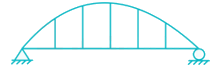Solution:

Ds = Dse + Dsi - R

Dse = External Indeterminacy

Dsi = Internal Indeterminacy

= 3 X No. of cuts required to make stable cantilever (C)

R = No. of Reactions added unnecessarily to make stable cantilever

Calculations:-

Dse = (2 + 1) - 3 = 0

Dsi = 3C = 3× 7 = 21

R = 1 (for hinge support) + 2 (roller support)

∴ Ds = 0 + 21 - 3 = 18

QUESTION: 7

In a two-hinged parabolic arch (consider arch to be initially unloaded) an increase in temperature induces -

Solution:

As loading is UDL and the shape of the arch is parabolic so there will be no moment in the arch rib.

But as the temperature increases in a two hinged arch (degree of indeterminacy one), the horizontal thrust will increase.

Moment due to horizontal thrust is – Py.

So maximum bending moment will be at crown as crown has highest value of y.

*Answer can only contain numeric values
QUESTION: 8

Find the maximum tension in the cable in KN if a unit load of 10 KN can move in either direction in the beam AB?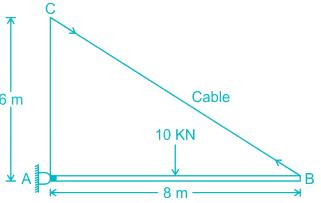Solution:

Let the 10 KN load is at a distance x from support A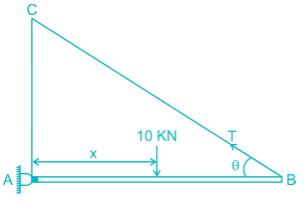T sin θ × 8 = 10x
⇒ T=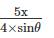So ‘T’ will be maximum when ‘x’ becomes maximum i.e; x = 8 m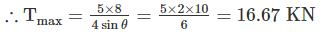*Answer can only contain numeric values
QUESTION: 9

A uniformly distributed load of 80 kN per meter run of length 3 m moves on a simply support girder of span 10 m. The magnitude of the ratio of Maximum negative shear force to the maximum positive shear force at 4 m from the left end will be _______ (up to two decimal places).

Solution:

For maximum positive shear force at C: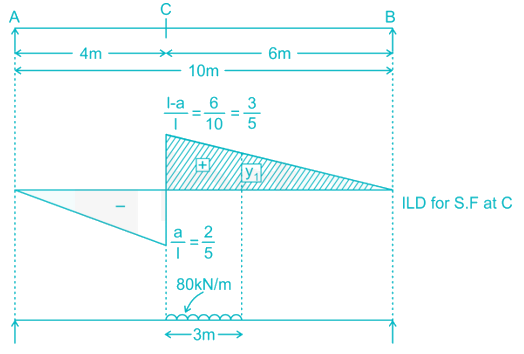Using Muller Breslau Principle.

y13=356y13=356

Y1 = 0.3

Maximum positive shear force at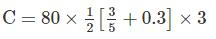(S.F)max1 =108kN

For maximum negative shear force at C: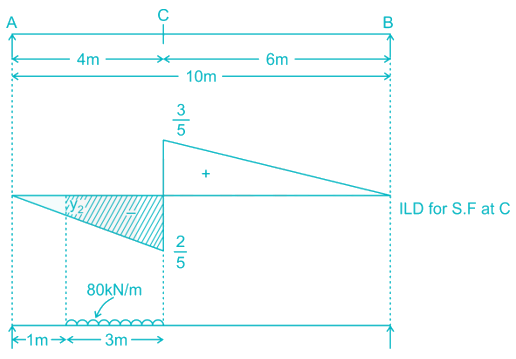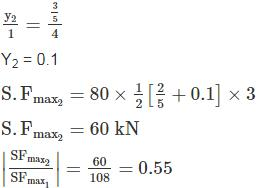*Answer can only contain numeric values
QUESTION: 10

Find the maximum reaction developed at B when a udl of 3KN/m of span 5m is moving towards right in the beam shown below:-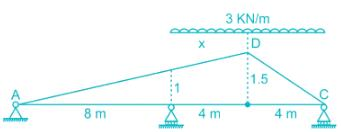Solution:

The influence line diagram for the reaction at B is shown below:-